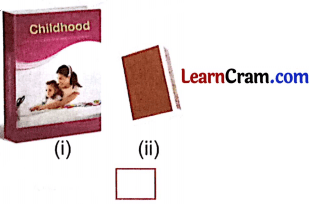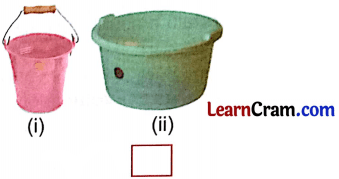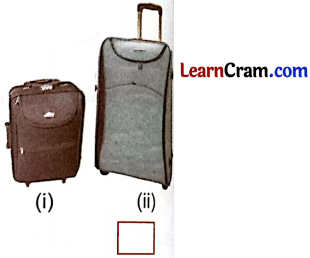# DAV Class 4 Maths Chapter 13 Worksheet 1 Solutions

The DAV Maths Class 4 Solutions and DAV Class 4 Maths Chapter 13 Worksheet 1 Solutions of Volume offer comprehensive answers to textbook questions.

## DAV Class 4 Maths Ch 13 WS 1 Solutions

Question 1.
Which of the following has more volume?

(a) Cricket ball or table tennis ball?
Solution:
Cricket ball

(b) Lemon or watermelon?
Solution:
Watermelon

(c) $$\frac{1}{2}$$ 1 polypack of milk or 1 1 polypack of milk?
Solution:
1 1 polypack of milk.Question 2.
In each of the following pairs, which object has lesser volume?

(a)Solution:
(ii) match box.

(b)Solution:
(i) bucket.

(c)Solution:
(i) small bag.Question 3.
Which of the following are true?

(a) A cricket ball and a football have the same volume.
Solution:
False

(b) An empty chalk box has less volume than a chalk box of same size and shape full of chalks.
Solution:
False

(c) Two oil cans of same size, one empty and one full of oil have the same volume.
Solution:
True.### DAV Class 4 Maths Chapter 13 Worksheet 1 Notes

• The measure of space a solid occupies is called the volume of the solid.
• Empty or full objects of same size and shape have the same volume.
• Volume of big sized object is more.
• Volume of cuboid = Length × Breadth × Height.
• Volume of cube = Edge × Edge × Edge.